# Mirrors, Polaroid Films, Light/Wavelength help needed

:(:(:( Please ease my aching soul by helping me with this...
I submitted this assignment online and I don't know the answers yet or however many I got wrong.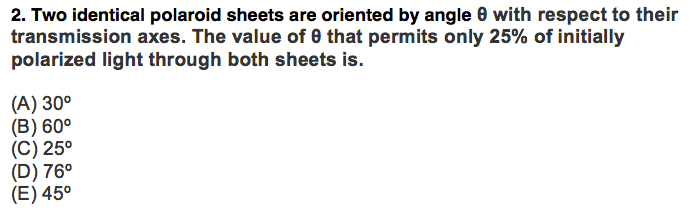I chose 45 because I know unpolarized light becomes this after going through a polarizer: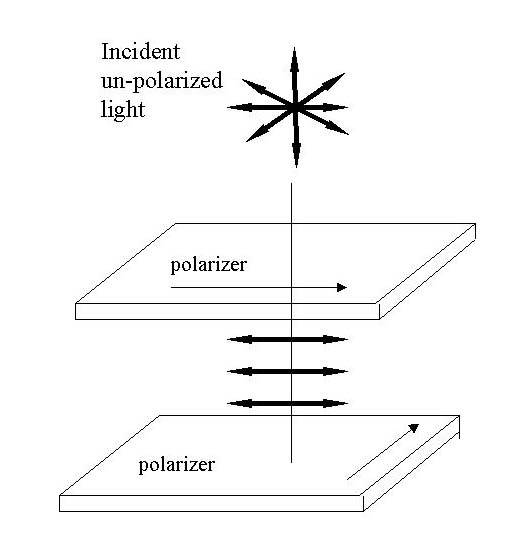That single line is 180º, so I did 0.25 x 180º = 45º.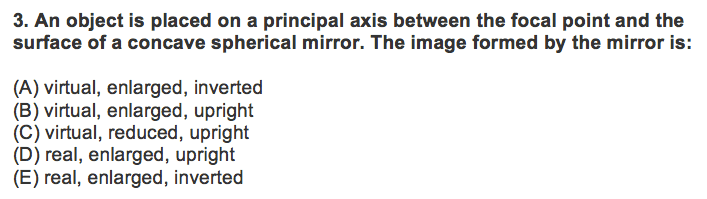I chose E…but after seeing this diagram below just now, I think I got the answer wrong and that it's supposed to be B… Is E not the answer?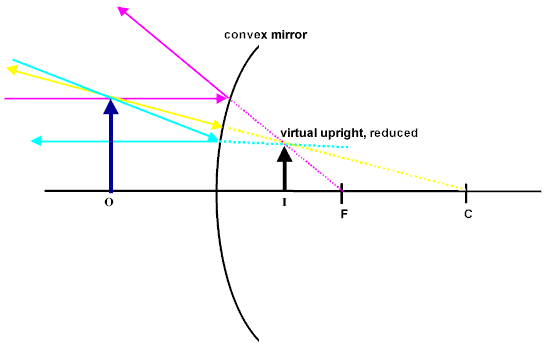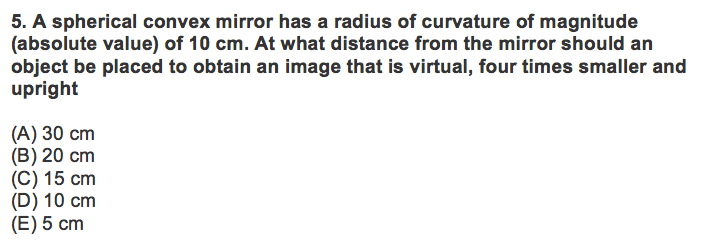I chose D…but I don't think it's right…
I did this:

Given:
R = 10 cm
f = -R/2 (since it's convex) = -10/2 = -5
do = ?
M = +4 x (image is upright)
di = -? (image is virtual)

M = -di/do
4 = -di/do
di = -4do

1/f = 1/do + 1/di
1/(-5) = 1/do + 1/(-4do)
-0.2 = 1/do - 1/4do
-0.2 = (4/4)1/do - (1/1)1/4do
-0.2 = (4 - 1)/4do
-0.2 = 3/4do
4do = 3/(-0.2)
4do = -0.6
do = -0.6/4
do = -0.15

Is the answer C? (I chose D originally and I can't change my answer….but now that i've worked it out step by step i think the answer is C)

mfb
Mentor
That single line is 180º, so I did 0.25 x 180º = 45º.
It is not, and there is no reason to multiply 180° by anything. Using the same argument, 90° would let 50% pass through, which is obviously wrong.

You can analyze this with polarization vectors and projetions on the polarization axis, or with existing formulas (Wikipedia, book, ...).

I did this:
Your result is very small (0.15 cm), there has to be some error in the calculation. I found one here:
4do = 3/(-0.2)
4do = -0.6

It is not, and there is no reason to multiply 180° by anything. Using the same argument, 90° would let 50% pass through, which is obviously wrong.

You can analyze this with polarization vectors and projetions on the polarization axis, or with existing formulas (Wikipedia, book, ...).

Your result is very small (0.15 cm), there has to be some error in the calculation. I found one here:

So basically all three of them are wrong?

It is not, and there is no reason to multiply 180° by anything. Using the same argument, 90° would let 50% pass through, which is obviously wrong.

You can analyze this with polarization vectors and projetions on the polarization axis, or with existing formulas (Wikipedia, book, ...).

Your result is very small (0.15 cm), there has to be some error in the calculation. I found one here:

Also, what about question number 3?

:( :(

mfb
Mentor
Also, what about question number 3?
Take a spoon and test it.
The graph below is not a concave mirror.

Take a spoon and test it.
The graph below is not a concave mirror.

Ok but my answer is still wrong though...
The answer for 3 would have to be D.

mfb
Mentor
Did you take the right ("inner") side of the spoon?

Also, what about question number 3?

Think about which side of the concave surface the image lies in.

If it lies on the left (the same side as where the object resides), it is real. If on the right, then the image is virtual. The focal equation is based on the reference of the surface of the mirror. Positive distance means real and negative distance means virtual.

Did you take the right ("inner") side of the spoon?

What do you mean?

mfb
Mentor
I don't know what is unclear about that question.
Like this, but with an object closer to the mirror.

I don't know what is unclear about that question.
Like this, but with an object closer to the mirror.

real (image on front), enlarged, inverted.

real (image on front), enlarged, inverted.

Not for an object lying inside of the focal point.

Why don't you try drawing out a a simple ray diagram?

mfb
Mentor
I did not find an image of an object closer to the focal point. The inversion is not there in your problem.

Not for an object lying inside of the focal point.

Why don't you try drawing out a a simple ray diagram?

I forgot how to because I did this back in high school and my teacher (in college) just gave us a simple chart to follow and didn't emphasize the fact that we had to draw a ray diagram. :(

Last edited:
I did not find an image of an object closer to the focal point. The inversion is not there in your problem.

The answer has to be B then...

Ok well since I know I got those top 3 questions wrong...I wouldn't mind moving on to the other questions I wouldn't mind you guys checking for me...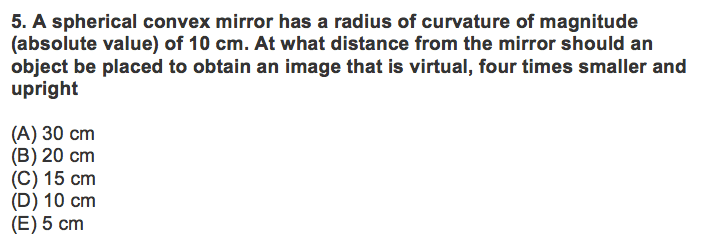I chose D…but I don't think it's right…
I did this:

Given:
R = 10 cm
f = -R/2 (since it's convex) = -10/2 = -5
do = ?
M = +4 x (image is upright)
di = -? (image is virtual)

____________________________

M = -di/do
4 = -di/do
di = -4do

____________________________

1/f = 1/do + 1/di
1/(-5) = 1/do + 1/(-4do)
-0.2 = 1/do - 1/4do
-0.2 = (4/4)1/do - (1/1)1/4do
-0.2 = (4 - 1)/4do
-0.2 = 3/4do
4do = 3/(-0.2)
4do = -0.6
do = -0.6/4
do = -0.15

Is the answer C? (I chose D originally and I can't change my answer….but now that i've worked it out step by step i think the answer is C)

The answer has to be B then...

Ok well since I know I got those top 3 questions wrong...I wouldn't mind moving on to the other questions I wouldn't mind you guys checking for me...I chose D…but I don't think it's right…
I did this:

Given:
R = 10 cm
f = -R/2 (since it's convex) = -10/2 = -5
do = ?
M = +4 x (image is upright)
di = -? (image is virtual)

____________________________

M = -di/do
4 = -di/do
di = -4do

____________________________

1/f = 1/do + 1/di
1/(-5) = 1/do + 1/(-4do)
-0.2 = 1/do - 1/4do
-0.2 = (4/4)1/do - (1/1)1/4do
-0.2 = (4 - 1)/4do
-0.2 = 3/4do
4do = 3/(-0.2)
4do = -0.6
do = -0.6/4
do = -0.15

Is the answer C? (I chose D originally and I can't change my answer….but now that i've worked it out step by step i think the answer is C)

Your magnification is wrong. It's 4x smaller, which means ##\frac{d_i}{d_o}## should be less than one since ##\frac{h_i}{h_o}## is reduced.

Your magnification is wrong. It's 4x smaller, which means ##\frac{d_i}{d_o}## should be less than one since ##\frac{h_i}{h_o}## is reduced.

Oh goodness...
So what should M equal?

Oh goodness...
So what should M equal?

Well, it's 4x smaller so what do you think?

I'm sure you can figure this out. Other than that there seems to be nothing else wrong with your calculations.

mfb
Mentor
Other than that there seems to be nothing else wrong with your calculations.
Well, there is a calculation error in the first post, I mentioned it in post 2:
Your result is very small (0.15 cm), there has to be some error in the calculation. I found one here:
4d0 = 3/(-0.2)
4d0 = -0.6